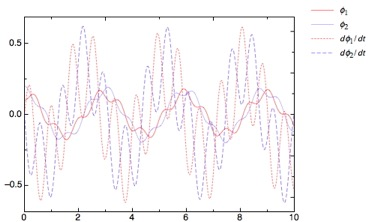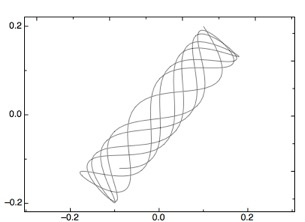## ODE ModuleODE Module is a pro Fit module. It solves initial value problems of sets of coupled first-order differential equations (up to 14). The solutions are plotted over the integration variable and against each other (phase diagrams). For information on the scientific analysis package pro Fit, visit the QuantumSoft pro Fit site. ODE Module is Freeware.

How It Works
Ordinary differential equations (ODEs) of any order can be reduced to a set of first-order differential equations which can be numerically integrated. The ODEs can be coupled as well. Consider a second-order ODE of the function x(t) like

x'' + q(t) x' = r(t) ,

where x' denotes the first and x'' the second derivative of x(t) with respect to t. Rename the first derivative to

y = x'

and rewrite the ODE like

y' = r(t) - q(t) y .

Now you have a set of two first-order differential equations:

x' = y
y' = r(t) - q(t) y

Starting from any initial point t0, where x and y (that is, x') are known, one can calculate the derivatives of x and y and thus integrate to a second point t1, using a numerical integration method like Euler's, Runge-Kutta or the like.
For example, a third-order ODE results in three first-order differential equations, two second-order ODEs result in four equations, respectively. In order to be flexible for systems of different degrees, we use the following naming conventions in the ODE Module:

y1 = x, y2 = y, y3 = z, ...

Then we write the differential equations above in the form

y1' = y2
y2' = r(t) - q(t) y2

When using ODE Module to integrate such systems of differential equations, you have to supply a pro Fit function that calculates the values of the derivatives at a given point using this set of equations. ODE Module uses the Runge-Kutta method (fixed or variable stepsize) for integration and calls this function. For futher information on installing and using ODE Module, see the Readme file.

History
2.0

• Use of VAR parameters for better speed and stability (Be sure to update your "derivs" functions according to the included examples!)
1.5
• Version for pro Fit 7.0
1.4
• Version for pro Fit 6.1
• Mac OS X Universal (PPC and Intel)
• Default line thickness 0.5 pt instead of 0.25 pt
1.3
• Carbon version for pro Fit 5.6 up to 6.0
• Increased number of equations to 14
1.2.1
• Fixed bug with N > 6 (I didn't consider the limitation of the pro Fit InputBox function)
• Increased the number of equations to 12
• Solved problem with phase diagrams when a function is constant
1.2
• Added option to save the calculated data in a pro Fit data window. This is useful for subsequent calculations with pro Fit.
1.1
• First public release
Credits
The Runge-Kutta algorithms were implemented by Press, Teukolsky, Vetterling, Flannery: Numerical Recipes in C. Cambridge University Press. pro Fit is made by QuantumSoft, Switzerland.

Warranties